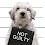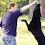## Wednesday, June 5, 2013

### Wednesday Brain Teaser 6-5-13

There is one four-digit whole number n, such that the last four digits of n^2 are in fact the original number n. What is n?

1.I don't think I am understanding the question

1.Fixed. It didn't like my HTML for the exponent.

2.I don't remember if Blogger would let you do n<sup>2</sup>...

Oddly, the comment-box won't let me put this Superscript code in as HTML, though it will let me use the HTML-codes for the angle-brackets.

On to the problem...I can re-write the description as an equation, with (n^2) equal to some integer that ends in 4 zeros, plus (n). But an integer ending in 4 zeros can be written as a smaller integer that's been multiplied by 10000.

n^2 = 10000*k + n

Another form of this equation is that
k = (n^2 - n) / 10000 = ( n*(n-1) )/10000

If (k) is an integer, then it looks like some factor of 10000 divides (n), and the remainder divides (n-1). But

10000 = (2^4)*(5^4) = 16*625

And only one of the numbers {n, n-1} can be even. Thus, if one of the numbers is divisible by 2, it must be divisible by 16. Similarly, the other number is not only divisible by 5, but by 625.

k = ( n*(n-1) ) / (16)*(625)

To move forward, let's assume (n) is divisible by 16, and (n-1) is divisible by 625.

Thus, there are some other integers (p) and (q) such that
(16*p) = n
(625*q) = (n-1)

which implies

n = (16*p) = (625*q) + 1

Not many multiples of 625 are 4-digit numbers. After a little testing, the only one that fits this equation is (625*15) = 9375, with 9375+1 = 9376 = (16*586). Thus,

n=9376

produces

n^2=87909376

(Confession: I brute-forced the answer of n=9376 using Excel...it's not too hard to set up a list of all 4-digit values of (n), check (n^2), and check the last 4 digits of (n^2). Then I looked at the result, thought a second, and told myself, "I can explain this result in a better way..."

In working through the solution above. Then I noticed that if I assumed that (n) was divisible by 625 instead of by 16, I can't find a 4-digit number that satisfies the question.

But 625 and 10625 both have squares that end in "0625", so I get a number that satisfies the equation (n^2) = (10000*k) + (n)...

What a way to spend a lunch-hour.)